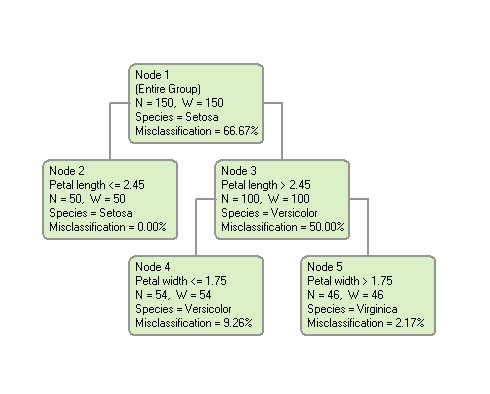# Introduction to Decision Trees

A decision tree is a logical model represented as a binary (two-way split) tree that shows how the value of a target variable can be predicted by using the values of a set of predictor variables. An example of a decision tree is shown below:The rectangular boxes shown in the tree are called “nodes”. Each node represents a set of records (rows) from the original dataset. Nodes that have child nodes (nodes 1 and 3 in the tree above) are called “interior” nodes. Nodes that do not have child nodes (nodes 2, 4 and 5 in the tree above) are called “terminal” or “leaf” nodes. The topmost node (node 1 in the example) is called the “root” node. (Unlike a real tree, decision trees are drawn with their root at the top). The root node represents all of the rows in the dataset.

A decision tree is constructed by a binary split that divides the rows in a node into two groups (child nodes). The same procedure is then used to split the child groups. This process is called “recursive partitioning”. The split is selected to construct a tree that can be used to predict the value of the target variable.

The name of the predictor variable used to construct a node is shown in the node box below the node number. For example, nodes 2 and 3 were formed by splitting node 1 on the predictor variable “Petal length”. If the splitting variable is continuous, the values going into the left and right child nodes will be shown as values less than or greater than some split point. The split point is 2.45 in this example. Node 2 consists of all rows with the value of “Petal length” less than or equal to 2.45, whereas node 3 consists of all rows with Petal length greater than 2.45. If the splitting variable is categorical, the categories of the splitting variable going into each node will be listed.

Tree Building Process

The first step in building a decision tree is to collect a set of data values that DTREG can analyze. This data is called the "learning" or "training" dataset because it is used by DTREG to learn how the value of a target variable is related to the values of predictor variables. This dataset must have instances for which you know the actual value of the target variable and the associated predictor variables. You might have to perform a study or survey to collect this data, or you might be able to obtain it from previously-collected historical records.

Once you obtain enough data for the learning dataset, this data is fed into DTREG which performs a complex analysis on it and builds a decision tree that models the data.

Using a Decision Tree

Once DTREG has created a decision tree, you can use it in the following ways:

• You can use the tree to make inferences that help you understand the “big picture” of the model. One of the great advantages of decision trees is that they are easy to interpret even by non-technical people. For example, if the decision tree models product sales, a quick glance might tell you that men in the South buy more of your product than women in the North. If you are developing a model of health risks for insurance policies, a quick glance might tell you that smoking and age are important predictors of health.
• You can use the decision tree to identify target groups. For example, if you are looking for the best potential customers for a product, you can identify the terminal nodes in the tree that have the highest percentage of sales, and then focus your sales effort on individuals described by those nodes.
• You can predict the target value for specific cases where you know only the predictor variable values. This is known as “scoring”.